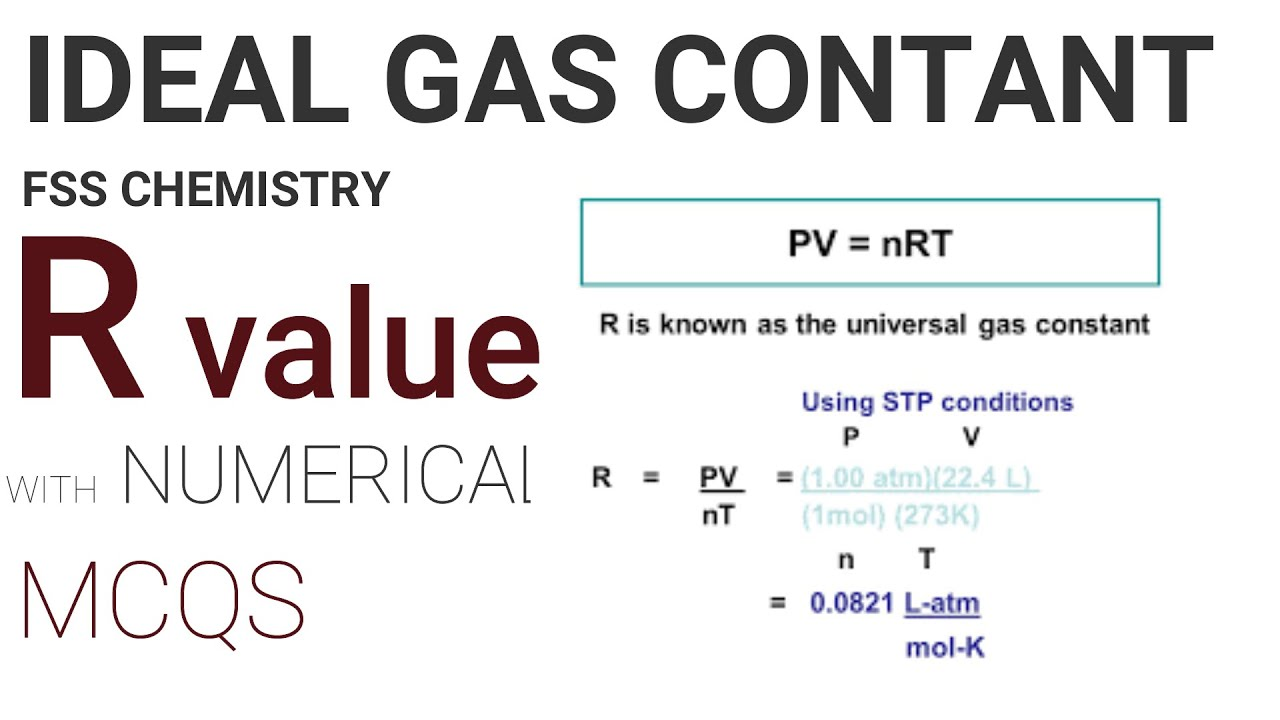# What Does R Stand For In Chemistry?

In chemistry and physics equations, “R” represents the gas constant, molar gas constant, general gas constant, or universal gas constant. A proportionality factor links energy scales and temperature scales in multiple equations.

## Gas Constant in Chemistry

• The gas constant has numerous names in chemistry, including the ideal gas constant and universal gas constant.
• It is the molar equivalent of the Boltzmann constant.
• The SI value for the gas constant is precisely 8.31446261815324 JK1mol. Typically, the decimal is rounded to 8.314The Gas Constant is the physical constant in the Ideal Gas Law equation:

PV= nRT

P is pressure, V is volume, n is the number of molecules, and T is temperature. By rearranging the equation, R may be determined:

R equals PV/nT

The gas constant is also included in the Nernst equation linking the reduction potential of a half-cell to the standard electrode potential:

E = E0 – (RT/nF).

E is the cell potential, E0 is the standard cell potential, R is the gas constant, T is the temperature, n is the number of moles of electrons being exchanged, F is Faraday’s constant, and Q is the reaction quotient.

The gas constant is equal to the Boltzmann constant but represented in terms of energy per temperature per mole. In contrast, the Boltzmann constant is written in terms of energy per temperature per molecule.

Physically, the gas constant is a proportionality constant that relates the energy scale to the temperature measurement for a mole of particles at a particular temperature.

The units for the gas constant vary based on the other units used in the formula.

## Why Is R Used for the Gas Constant?

Some individuals believe that the sign R is chosen for the gas constant in honor of the French chemist Henri Victor Regnault, whose experiments were utilized to calculate the constant for the first time.

However, it is uncertain if his name is the origin of the convention used to represent the constant.

View Also :

What Is ATM In Chemistry?

What Are Tassels In Biology Class 12?

What Is Cell In Physics?

What Is Rest In Physics?

How One Worlds Oldest Science Experiments